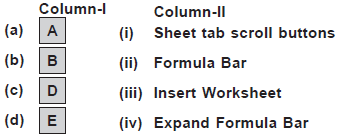# User Forum

Subject :NCO    Class : Class 7

Match the following.A a -(ii), b-(iii), c -(iv), d-(i)
B a -(i), b-(ii), c-(iv), d -(iii)
C a -(iv), b-(i), c-(ii), d -(iii)
D a -(iii), b -(iv), c-(i), d-(ii)

what does this question mean?